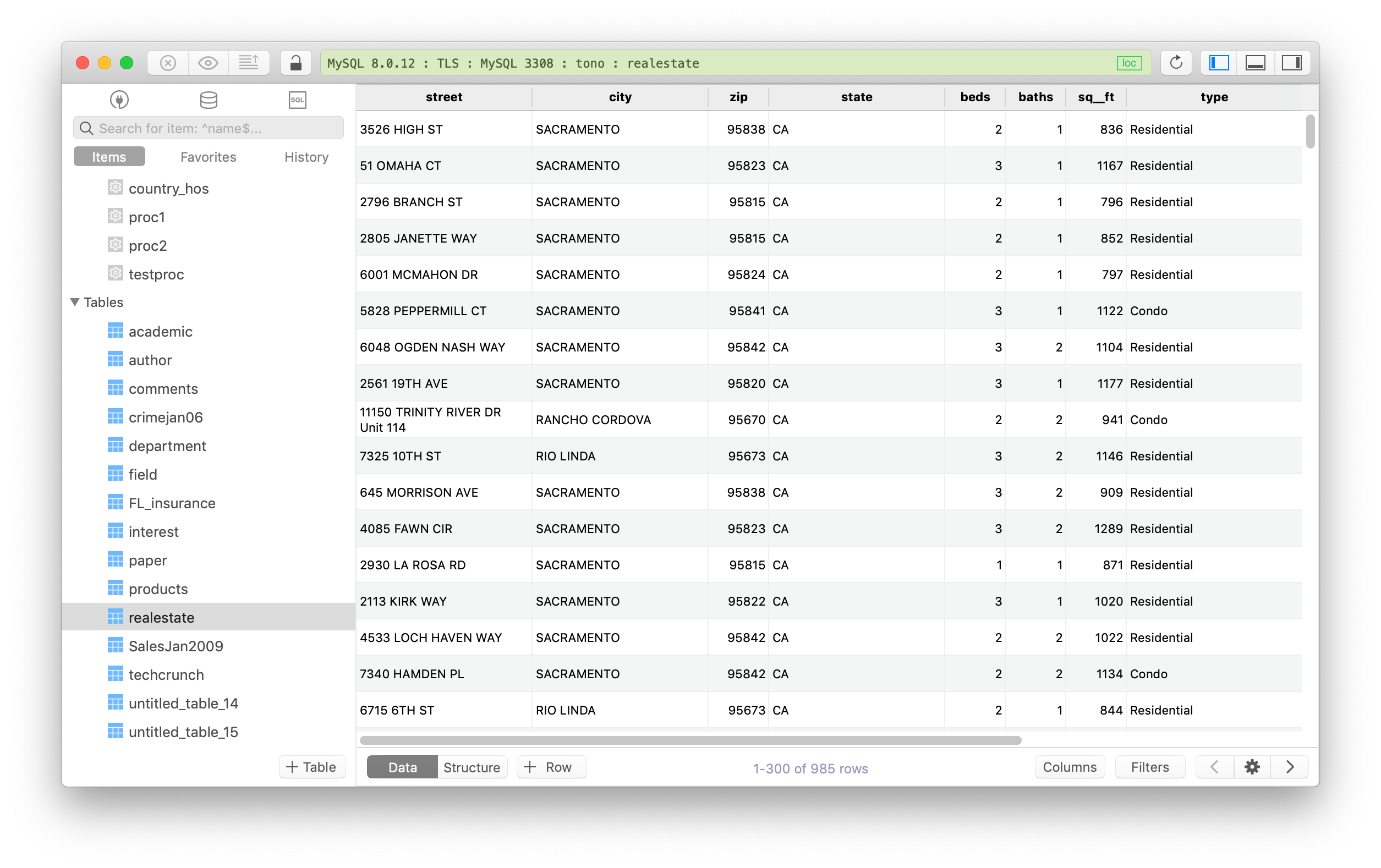#### A. Data Definition Language (DDL)

1. CREATE

`CREATE` queries are used to create a new database or table.

``````CREATE TABLE table_name (
column_1 datatype_1,
column_2 datatype_2,
);
``````

2. ALTER

`ALTER` queries are used to modify the structure of a database or a table such as adding a new column, change the data type, drop, or rename an existing column, etc.

``````ALTER TABLE table_name
``````

3. DROP

`DROP` queries are used to delete a database or table. You should also be careful when using this type of query because it will remove everything, including table definition along with all the data, indexes, triggers, constraints and permission specifications for that table.

``````DROP TABLE table_name;
``````

4. TRUNCATE

`TRUNCATE` queries are used to clean the table, remove all the existing records, but not the table itself.

``````TRUNCATE TABLE table_name;
``````

#### B. Data Manipulation Language (DML): managing data within table object.

1. SELECT

• `SELECT … FROM …` is the most basic and commonly used query in SQL. It’s used for retrieving data from a table.

A common `SELECT` query is broken down into four main parts:

• SELECT
• FROM
• WHERE
• ORDER BY

Let’s look deeper

• To see data of an entire table:
``````SELECT * FROM table_name;
``````
• To see data in some specific columns:
``````SELECT column_name(s) FROM table_name;
``````
• To see data from your table based on some conditions, this is the case for `WHERE` to be used:
``````SELECT column_name(s)
FROM table_name
WHERE condition(s);
``````

By using `WHERE` in a `SELECT` query, we add one or more conditions and restrict the number the records affected by the query.

Or in other words, it’s being a filter to filter out only the records that match the conditions as the result.

Example:

``````SELECT * FROM students
WHERE state_code = 'CA'
``````

That query to show every record from the table students that match the state_code “CA”.

• `ORDER BY` is a clause that indicates you want to sort the result set by a particular column either alphabetically or numerically.
``````SELECT column_name
FROM table_name
ORDER BY column_name ASC | DESC;
``````

2. INSERT

`INSERT INTO` queries are used to insert one or more rows of data (new records) into an existing table.

``````INSERT INTO table_name (column_1, column_2, column_3, ...)
VALUES (value_1, value_2, value_3, ...);
``````

Example:

``````INSERT INTO students (full_name, student_id, state_code)
VALUES (“Alex Jonas”, 234, "CA");
``````

3. UPDATE

`UPDATE` queries are used to modify an existing table and update it with new data based on some conditions.

``````UPDATE table_name
SET column_1 = value_1, column_2 = value_2, ...
WHERE condition;
``````

4. DELETE

`DELETE FROM` queries are used to remove the records from a table based on some conditions. `DELETE FROM` is similar to `TRUNCATE` but it limits the number of rows being affected by the query using the conditions.

``````DELETE FROM table_name
WHERE condition;
``````

#### C. Aggregate Functions

• `AVG()` returns the average value for a numeric column.
``````SELECT AVG(column_name)
FROM table_name;
``````
• `SUM()` returns the sum of all the values in a column.
``````SELECT SUM(column_name)
FROM table_name;
``````
• `ROUND()` rounds the values in the column to the number of decimal places specified by the integer.
``````SELECT ROUND(column_name, integer)
FROM table_name;
``````
• `MAX()` returns the largest value in a column.
``````SELECT MAX(column_name)
FROM table_name;
``````
• `MIN()` returns the smallest value in a column.
``````SELECT MIN(column_name)
FROM table_name;
``````
• `COUNT()` counts the number of rows where the column is not NULL.
``````SELECT COUNT(column_name)
FROM table_name;
``````

#### D. Additional clauses and functions

• You can use `AS` to rename a column or table temporarily using an alias on the result view.
``````SELECT column_name AS 'Alias'
FROM table_name;
``````
• The `BETWEEN` operator is used to select the value within a certain range.
``````SELECT column_name(s)
FROM table_name
WHERE column_name BETWEEN value_1 AND value_2;
``````
• `GROUP BY` is a clause in SQL that is only used with aggregate functions (COUNT, MAX, MIN, SUM, AVG). It is used in collaboration with the SELECT statement to arrange identical data into groups.
``````SELECT column_name, COUNT(*)
FROM table_name
GROUP BY column_name;
``````
• `HAVING` is used to replace `WHERE` to work with aggregate functions. `WHERE` clause introduces a condition on individual rows; `HAVING` clause introduces a condition on aggregations.

`HAVING` is typically used with `GROUP BY`.

``````SELECT column_name, COUNT(*)
FROM table_name
GROUP BY column_name
HAVING COUNT(*) > value;
``````
• `IS NULL` and `IS NOT NULL` are used to test whether a column value is empty or not.
``````SELECT column_name(s)
FROM table_name
WHERE column_name IS NULL;
``````
• `LIKE` is a special operator used with the WHERE clause to search for a specific pattern in a column.
``````SELECT column_name(s)
FROM table_name
WHERE column_name LIKE pattern;
``````
• You can use `LIMIT` to specify the maximum number of records you want to show in a result set.
``````SELECT *
FROM table_name
LIMIT number;
``````
• `OR` is used to combine two or more conditions in a where clause. The results have to match at least one of the conditions specified.
``````SELECT *
FROM table_name
WHERE condition_1
OR condition_2;
``````
• `SELECT DISTINCT` returns unique values in the specified column(s).
``````SELECT DISTINCT column_name
FROM table_name;
``````
• An `OUTER JOIN` will combine rows from different tables even if the join condition is not met. Every row in the left table is returned in the result set, and if the join condition is not met, then NULL values are used to fill in the columns from the right table.
``````SELECT column_name(s)
FROM table_1
LEFT JOIN table_2
ON table_1.column_name = table_2.column_name;
``````
• An `INNER JOIN` will combine rows from different tables if the join condition is true.
``````SELECT column_name(s)
FROM table_1
JOIN table_2
ON table_1.column_name = table_2.column_name;
``````

Need a good tool for learning and practising SQL? Try TablePlus.

It’s modern, native tool with elegant GUI that allows you to simultaneously manage multiple databases such as MySQL, PostgreSQL, SQLite, Microsoft SQL Server and more.  It’s free anyway.